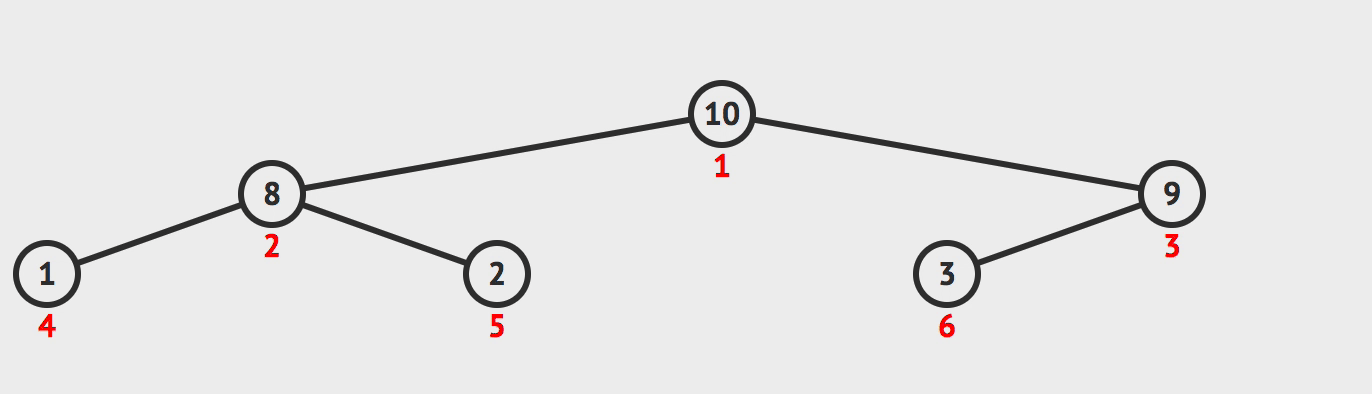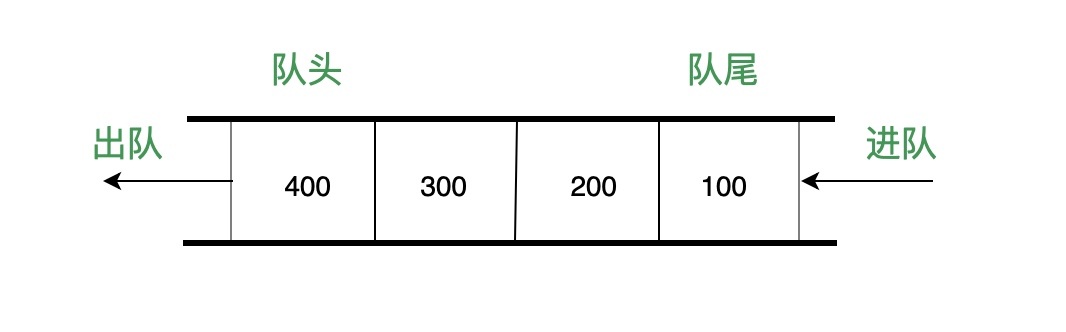# 来自大厂 300+ 道前端面试题大全附答案（整理版）+前端常见算法面试题~~最全面详细前端 同时被 2 个专栏收录30 篇文章 8 订阅

“全方位总结一下所遇到的面试题目，与大家共同学习，也是对自己的一次总结”

### 前言

#### 前端开发所需掌握知识点概要：

HTML&CSS：浏览器内核、渲染原理、依赖管理、兼容性、CSS语法、层次关系，常用属性、布局、选择器、权重、CSS盒模型、Hack、CSS预处理器、CSS3动画

JavaScript：

ajax、jQuery、Bootstrap、axios、Vue、Vuex、React、element-ui、layui、webpack#### 1、HTML面试题

• html 语义化
• canvas 相关
• svg和canvas的区别？
• html5有哪些新特性？
• 如何处理HTML5新标签的浏览器兼容问题？
• 说说 title 和 alt 属性
• HTML全局属性(global attribute)有哪些#### 2、CSS面试题

• 让一个元素水平垂直居中，到底有多少种方案？
• 浮动布局的优点？有什么缺点？清除浮动有哪些方式？
• 使用display:inline-block会产生什么问题？解决方法？
• 布局题：div垂直居中，左右10px，高度始终为宽度一半
• 盒模型
• CSS如何进行品字布局？
• CSS如何进行圣杯布局
• CSS如何实现双飞翼布局？
• 什么是BFC？
• 什么是 Css Hack？ie6,7,8 的 hack 分别是什么？
• 描述一个”reset”的 CSS 文件并如何使用它。知道 normalize.css 吗？你 了解他们的不同之处？
• CSS 中 link 和@import 的区别是？
• 为什么要初始化样式？#### 3、JavaScript面试题

• 0.1+0.2为什么不等于0.3？
• 什么是BigInt?
• 为什么需要BigInt?
• 什么是闭包？
• 闭包产生的原因?
• 闭包有哪些表现形式?
• 原型对象和构造函数有何关系？
• 能不能描述一下原型链？
• JS如何实现继承？
• null是对象吗？为什么？
• call 和 apply 的区别
• 描述一下 V8 执行一段JS代码的过程？
• 关于JS中一些重要的api实现
• == 和 ===有什么区别？
• 如何让if(a == 1 && a == 2)条件成立？#### 4、前端框架面试题

Vue

• Vue 双向绑定原理
• 描述下 vue 从初始化页面–修改数据–刷新页面 UI 的过程？
• 你是如何理解 Vue 的响应式系统的?
• 组件中写 name 选项有什么作用？
• vue 中怎么重置 data?
• vue 首屏加载优化
• vuex是什么？怎么使用？哪种功能场景使用它？
• vuex有哪几种属性？
• 虚拟 DOM 实现原理
• Vue 中 key 值的作用？
• 什么是MVVM？
• mvvm和mvc区别？它和其它框架（jquery）的区别是什么？哪些场景适合？React

• React有什么特点？
• 列出React的一些主要优点。
• React有哪些限制？
• 什么是JSX？
• 你了解 Virtual DOM 吗？解释一下它的工作原理。
• 与 ES5 相比，React 的 ES6 语法有何不同？
• 解释 React 中 render() 的目的。
• React中的状态是什么？它是如何使用的？
• 如何更新组件的状态？
• 如何模块化 React 中的代码？
• React中的事件是什么？
• 如何在React中创建一个事件？
• 你对 React 的 refs 有什么了解？
• 列出一些应该使用 Refs 的情况。
• 如何在 React 中创建表单
• 什么是高阶组件（HOC）？
• MVC框架的主要问题是什么？
• Redux与Flux有何不同？
• 数据如何通过 Redux 流动？
• 什么是React 路由？
• 为什么React Router v4中使用 switch 关键字 ？#### 5、浏览器面试题

• 能不能说一说浏览器缓存?
• 能不能说一说浏览器的本地存储？各自优劣如何？
• 能不能实现事件的防抖和节流？
• 浏览器缓存
• 谈谈你对重绘和回流的理解
• 能不能实现图片懒加载？
• 说一说从输入URL到页面呈现发生了什么？
• 能不能说一说XSS攻击？
• BOM对象模型#### 6、计算机网络面试题

• HTTP 缓存
• HTTP 常用的状态码及使用场景？
• HTTP 常用的请求方式，区别和用途？
• 你对计算机网络的认识怎么样
• HTTPS 是什么？具体流程
• WebSocket与Ajax的区别
• TCP 如何保证有效传输及拥塞控制原理。
• TCP 协议怎么保证可靠的，UDP 为什么不可靠？

#### 7、一些开放性题目

1)说说最近最流行的一些东西吧？常去哪些网站？

2)自我介绍：除了基本个人信息以外，面试官更想听的是你与众不同的地方和你的优势。

3)项目介绍

4)前端开发的职业现状和前景是什么？

5)平时是如何学习前端开发的？

6)1-3年工作经验，你有信心顺利跳槽到BATJ等一线互联网大公司吗？

7)你觉得哪个框架比较好,好在哪里

8)你觉得最难得技术难点是什么

#### 8、算法题

##### 链表

var reverseList = function(head) {
// 判断下变量边界问题
// 初始设置为空，因为第一个节点反转后就是尾部，尾部节点指向 null
let pre = null
let next
// 判断当前节点是否为空
// 不为空就先获取当前节点的下一节点
// 然后把当前节点的 next 设为上一个节点
// 然后把 current 设为下一个节点，pre 设为当前节点
while(current) {
next = current.next
current.next = pre
pre = current
current = next
}
return pre
};

##### 二叉树遍历
• 原理: 递归
function traversal(node,tempOrderTraversal) {
if (node != null) {
// tempOrderTraversal.push(node.value) 前序遍历
if (node.left != null) {
preOrderTraversal(node.left,tempOrderTraversal)
}
// tempOrderTraversal.push(node.value) 中序遍历
if (node.right != null) {
preOrderTraversal(node.right,tempOrderTraversal)
}
// tempOrderTraversal.push(node.value) 后序遍历
}
}


// 非递归遍历
var kthSmallest = function(root, k) {
const tempArr = [];
let result;
tempArr.push(root);
while (tempArr.length > 0) {
result = tempArr.pop();
if (result.value == k) break;
if (result.left != null) tempArr.push(result.left);
if (result.right != null) tempArr.push(result.right);
}
return result;
};

##### 堆排序

• 大根堆是某个节点的所有子节点的值都比他小
• 小根堆是某个节点的所有子节点的值都比他大

1. 首先遍历数组，判断该节点的父节点是否比他小，如果小就交换位置并继续判断，直到他的父节点
比他大
2. 重新以上操作 1，直到数组首位是最大值
3. 然后将首位和末尾交换位置并将数组长度减一，表示数组末尾已是最大值，不需要再比较大小
4. 对比左右节点哪个大，然后记住大的节点的索引并且和父节点对比大小，如果子节点大就交换位置
5. 重复以上操作 3 - 4 直到整个数组都是大根堆。以下是实现该算法的代码
function heap(array) {
checkArray(array);
// 将最大值交换到首位
for (let i = 0; i < array.length; i++) {
heapInsert(array, i);
}
let size = array.length;
// 交换首位和末尾
swap(array, 0, --size);
while (size > 0) {
heapify(array, 0, size);
swap(array, 0, --size);
}
return array;
}
function heapInsert(array, index) {
// 如果当前节点比父节点大，就交换
while (array[index] > array[parseInt((index - 1) / 2)]) {
swap(array, index, parseInt((index - 1) / 2));
// 将索引变成父节点
index = parseInt((index - 1) / 2);
}
}
function heapify(array, index, size) {
let left = index * 2 + 1;
while (left < size) {
// 判断左右节点大小
let largest =
left + 1 < size && array[left] < array[left + 1] ? left + 1 : left;
// 判断子节点和父节点大小
largest = array[index] < array[largest] ? largest : index;
if (largest === index) break;
swap(array, index, largest);
index = largest;
left = index * 2 + 1;
}
}


function insertSort(arr) {
if(arr == null  || arr.length <= 0){
return [];
}
var len = arr.length;
for(var i = 1; i < len; i++) {
for(var j = i - 1; j >= 0 && arr[j] > arr[j + 1]; j--) {
swap(arr, j, j + 1);
}
}
return arr;
}

function swap(arr, i, j){
var temp = arr[i];
arr[i] = arr[j];
arr[j] = temp;
}


let unique = function(arr) {
let hashTable = {};
let data = [];
for(let i=0,l=arr.length;i<l;i++) {
if(!hashTable[arr[i]]) {
hashTable[arr[i]] = true;
data.push(arr[i]);
}
}
return data
}
module.exports = unique;


def quick_sort(arr):
if len(arr) < 2:
return arr
mid = arr[len(arr)//2]
left, right = [], []
arr.remove(mid)
for item in arr:
if item > mid:
right.append(item)
else:
left.append(item)
return quick_sort(left)+[mid]+quick_sort(right)

b = [11, 99, 33, 69, 77, 88, 55, 11, 33, 36, 39, 66, 44, 22]
print(quick_sort(b))


var lengthOfLongestSubstring = function(s) {
let window = {};
let left = 0, right = 0;
let maxLen = 0, maxStr = '';
while (right < s.length) {
let c = s[right];
right++;
if (window[c]) window[c]++;
else window[c] = 1
while (window[c] > 1) {
let d = s[left];
left++;
window[d]--;
}
if (maxLen < right - left) {
maxLen = right - left;
}
}
return maxLen;
};function Queue() {
let items = []
this.enqueue = function(e) {
items.push(e)
}
this.dequeue = function() {
return items.shift()
}
this.isEmpty = function() {
return items.length === 0
}
this.front = function() {
return items
}
this.clear = function() {
items = []
}
this.size = function() {
return items.length
}
}#### ☀️ 由于篇幅原因，这里只能分享部分面试题截图，如有需要以上完整面试题宝典及答案PDF的，可以点击这里免费自取！！### 推荐教程：08-05
08-11302106-04534
08-10
04-30
05-065558
01-201421
10-05734
11-2463
06-03109
04-02
03-171016
09-143万+
10-0716万+
03-30728
07-26190
04-222万+
03-22251
02-21535
09-112627点击重新获取扫码支付余额充值